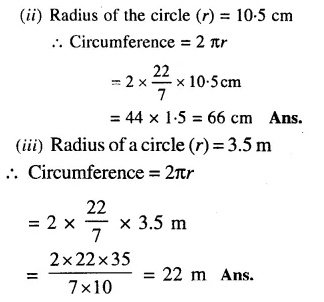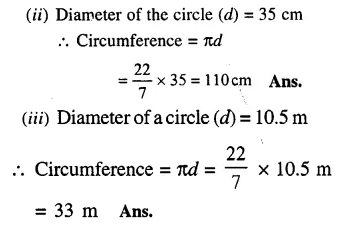## RS Aggarwal Class 6 Solutions Chapter 21 Concept of Perimeter and Area Ex 21B

These Solutions are part of RS Aggarwal Solutions Class 6. Here we have given RS Aggarwal Solutions Class 6 Chapter 21 Concept of Perimeter and Area Ex 21B

Other Exercises

Question 1.
Solution:
(i) Radius of the circle (r) = 28 cm
Circumference = 2 πr
= 2 x $$\\ \frac { 22 }{ 7 }$$ x 28 cm
= 176 cm Ans.Question 2.
Solution:
(i) Diameter of the circle (d) = 14 cm
Circumference = πd
= $$\\ \frac { 22 }{ 7 }$$ x 14
= 44 cmQuestion 3.
Solution:
Circumference of the circle = 176 cm
Let r be the radius, thenQuestion 4.
Solution:
Circumference of a wheel = 264 cm
Let d be its diameter, then
πd = 264
=> $$\\ \frac { 22 }{ 7 } d$$
= 264Question 5.
Solution:
Diameter of the wheel (d) = 77 cm
Circumference = πdQuestion 6.
Solution:
Diameter of the wheel = 70 cm
circumference = πdHope given RS Aggarwal Solutions Class 6 Chapter 21 Concept of Perimeter and Area Ex 21B are helpful to complete your math homework.

If you have any doubts, please comment below. Learn Insta try to provide online math tutoring for you.